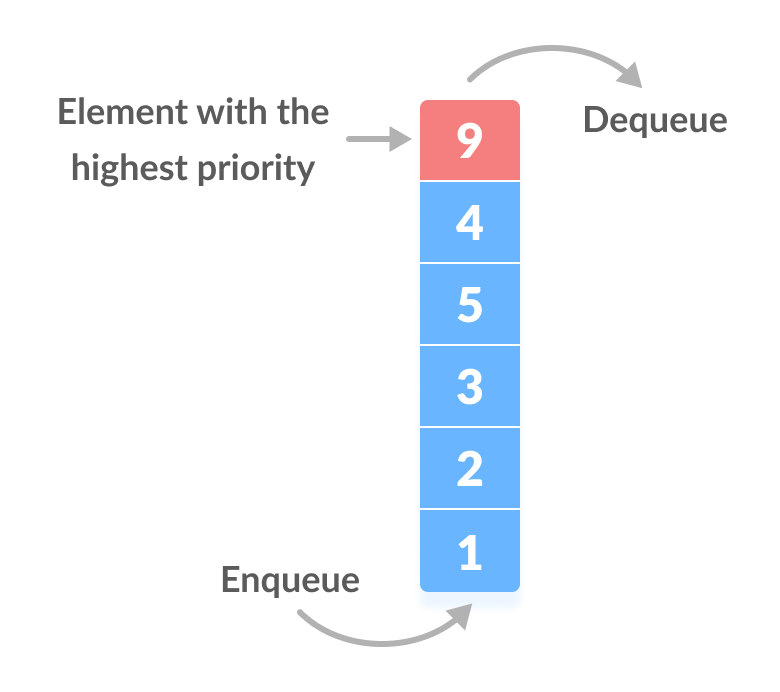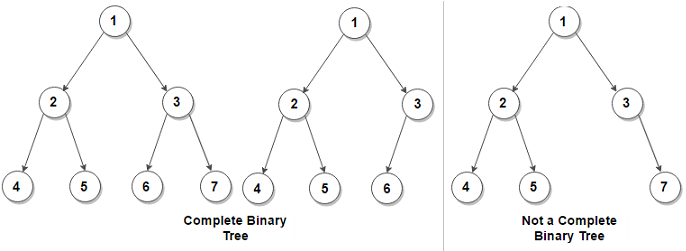# Priority Queue using C++

## Introduction

Priority Queue is quite similar to the data structure you are already familiar with, YES Queues. A priority queue is also a queue, but the magical thing here is that a priority queue keeps track of the most important item or the item with the highest priority.

Now just like a bag, you can add elements in the priority queue. However, the only element that can be accessed or removed is the one with the highest priority.Source: iq.opengenus

Okay, but what priority are we talking about? In most cases, the value of the element itself is considered for assigning the priority, i.e., its magnitude.

For example, The element whose magnitude is highest is considered to be the element with the highest priority. However, in other cases, we can also mark an element with the lowest value as the element with the highest priority.

Priorities can also be set by our needs. Thus we can classify them into two categories:

1. Min Priority Queue: Minimum element has the highest priority.
2. Max Priority Queue: Maximum element has the highest priority.

Now, this sounds very interesting, but you must be thinking about how to implement it. The answer is a heap data structure. Heaps? What are they?

A heap is a data structure based on trees where the root of the tree contains the element with the highest or the lowest priority. A heap is a complete binary tree, Another new word? Well, In a complete binary tree, every node has two child nodes except the leaf nodes. This means all the leaf nodes should be at the same level, and all other internal nodes should contain two child nodes each.Source: source

In the first image, all levels are filled, and each node except the leaf node has two children, whereas if we look at the second image we can see there are some nodes that don't have two children (Node with value 3).

Now let’s see how we can use the priority queue using c++ (STL)

## Priority Queue using C++ STL.

By default, a max priority queue is created in c++.

## Syntax for Min Priority Queue

Here, greater<int> is a functional object which is used for performing comparisons.

## Operations on Priority Queue

Let us see first how to insert elements in a priority queue.

To insert an element, we have a push() function. Let's see how it works.

### push()

Time Complexity of push() : O(logN), where ‘N’ is the size of the priority queue.

Okay, now you know how to insert an element in the priority queue but how to access the topmost element?

We can do that easily by the top() function provided in STL. Let’s understand it better using the following example.

### top()

Output

Time Complexity of top()O(1)

What if I have to delete the topmost element?

To achieve that, we can use the pop() function, as shown below:

### pop()

Output:

Time Complexity of pop(): O(logN), where ‘N’ is the size of the priority queue.

## Other Important Functions

By now, you have understood the basic functions of the priority queue using c++. Now let’s look at other functions that STL provides us.

1. empty() – It checks if the queue is empty or not. If the queue is empty, it returns true, otherwise false. It does not take any parameters.

Time Complexity of empty() : O(1)

2. size() – This function returns the number of elements present in the priority queue. It does not take any parameters.

Output

Time Complexity of size() : O(1)

3. swap() –   It is used to exchange the contents of two priority queues but the queues must be of the same type, although sizes may differ. It takes one parameter, i.e., the priority queue whose values need to be swapped.

Output

Time Complexity of swap() : O(N), where ‘N’ is the number of elements.

4. priority_queue :: value_type:  This method represents the type of object that is stored in our priority queue. It acts as a synonym for the template parameter.

Output

5. emplace() – The emplace() function adds a new element in a container at the top of the priority queue. It takes the value that needs to be added as a parameter.

Output

Time Complexity of emplace() : O(logN) , where ‘N’ is the size of the priority queue.

Q) What’s the difference between push and emplace?

In push() function, an object is created and then it is inserted into the priority_queue whereas, in the case of emplace(),  in-place object construction takes place which in turn saves creating an unnecessary copy.

## Key Takeaways

Now, you have mastered the basics of priority queue using C++; it’s time to move ahead to CodeStudio and practice some famous interview problems in the priority queue. CodeStudio offers interesting interview experiences and blogs like this one. Till then, keep learning, and Happy Coding!